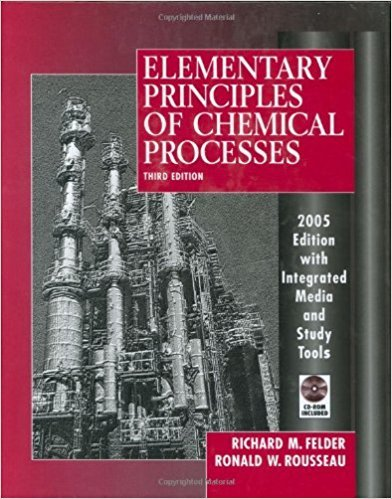×
Get Full Access to Elementary Principles Of Chemical Processes - 3 Edition - Chapter 4 - Problem 4.9
Get Full Access to Elementary Principles Of Chemical Processes - 3 Edition - Chapter 4 - Problem 4.9

×

# Strawberries contain about 15 wt% solids and 85 wt% water.ISBN: 9780471687573 143

## Solution for problem 4.9 Chapter 4

Elementary Principles of Chemical Processes | 3rd Edition

• Textbook Solutions
• 2901 Step-by-step solutions solved by professors and subject experts
• Get 24/7 help from StudySoup virtual teaching assistantsElementary Principles of Chemical Processes | 3rd Edition

4 5 1 319 Reviews
21
4
Problem 4.9

Strawberries contain about 15 wt% solids and 85 wt% water. To make strawberry jam, crushed strawberries and sugar are mixed in a 45:55 mass ratio, and the mixture is heated to evaporate water until the residue contains one-third water by mass. (a) Draw and label a flowchart of this process. (b) Do the degree-of-freedom analysis and show that the system has zero degrees of freedom (i.e., the number of unknown process variables equals the number of equations relating them). If you have too many unknowns, think about what you might have forgotten to do. (c) Calculate how many pounds of strawberries are needed to make a pound of jam.

Step-by-Step Solution:

Problem 4.9

Strawberries contain about 15 wt% solids and 85 wt% water. To make strawberry jam, crushed strawberries and sugar are mixed in a 45:55 mass ratio, and the mixture is heated to evaporate water until the residue contains one-third water by mass. (a) Draw and label a flowchart of this process. (b) Do the degree-of-freedom analysis and show that the system has zero degrees of freedom (i.e., the number of unknown process variables equals the number of equations relating them). If you have too many unknowns, think about what you might have forgotten to do. (c) Calculate how many pounds of strawberries are needed to make a pound of jam.

Step by step solution

Step 1 of 3

a)

The flow of the given process as follows.Here the mass fraction of solids (assume as sugar) in strawberries (of mass m.) is 0.15 and

the mass fraction of water in strawberries is 0.85.

And the mass fraction of sugar in strawberry jam (of mass 1.00 lb) is 0.667 and mass fraction of water in strawberries is 0.33.

____________________________________________________________________________

Step  2 of 3

b)

The number of degrees of freedom is given by equation:Here, unknowns are mass of strawberries ( m ), sugar (m,) and evaporated water (m.) that is

3. And mass balances for components sugar and water each can be written. Other equation

comes from the mass ratio of sugar to strawberry (which is known).

So, the number of degrees of freedom is= 3-(2+1)

=10

Therefore, the number of degrees of freedom is 10.

_____________________________________________________________________________

Step 3 of 3

c)

The mass ratio of strawberries to sugar in the initial mixture is 45:55. That is,Now, write the sugar mass balance equation.Substitutein above relation and solve form,Thus,strawberries are needed to makejam.

__________________________________________________________________________

Step 2 of 3

Step 3 of 3

##### ISBN: 9780471687573

This textbook survival guide was created for the textbook: Elementary Principles of Chemical Processes, edition: 3. Since the solution to 4.9 from 4 chapter was answered, more than 1578 students have viewed the full step-by-step answer. The full step-by-step solution to problem: 4.9 from chapter: 4 was answered by , our top Chemistry solution expert on 11/15/17, 02:42PM. This full solution covers the following key subjects: Water, strawberries, process, freedom, mass. This expansive textbook survival guide covers 13 chapters, and 710 solutions. Elementary Principles of Chemical Processes was written by and is associated to the ISBN: 9780471687573. The answer to “Strawberries contain about 15 wt% solids and 85 wt% water. To make strawberry jam, crushed strawberries and sugar are mixed in a 45:55 mass ratio, and the mixture is heated to evaporate water until the residue contains one-third water by mass. (a) Draw and label a flowchart of this process. (b) Do the degree-of-freedom analysis and show that the system has zero degrees of freedom (i.e., the number of unknown process variables equals the number of equations relating them). If you have too many unknowns, think about what you might have forgotten to do. (c) Calculate how many pounds of strawberries are needed to make a pound of jam.” is broken down into a number of easy to follow steps, and 109 words.

Unlock Textbook Solution